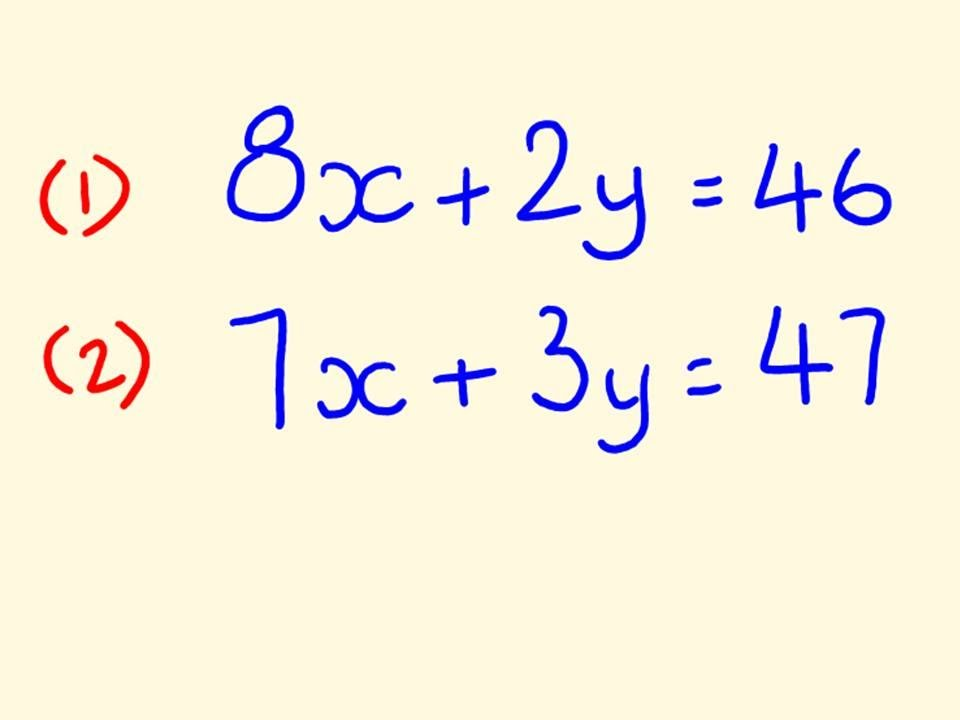Sign in# Can you solve this Mathematics equation simultaneously

Educational for grade 12 and 11

11:46

SundayCan you solve this simultaneous equation within 5 minute?

First of all, you need to know what do they mean simultaneously.

This simply means you have to calculate for the two unknown variables which is x and y.

For that you can not just solve them.

## Tips on how to get the right answer

•You use two methods, either elimination method or substitution method.

•With substitution method, you need to make one variable subject of the formula in order to get the new equation that would be substituted to either equation 1 or 2.

•Once you substituted, solve for one variable which could be x or y.

• After finding one answer, you can now simply substitute into into any other equation.

## Another method is Elimination

•You have to make sure that the equation are matching with their variables.

•This one is tricky, you have to multiply both equation by the first number of each equation then eliminate.

Now let's try to solve the one above, you can write it down then send your answers here under the comments.

Don't rush it or else you will get the wrong answer.

I'll drop the correct answer after 5PM

Content created and supplied by: Breaking-Now (via Opera News )